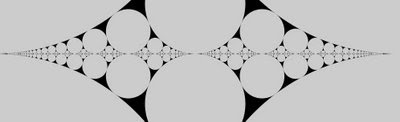## Wednesday, February 11, 2009

### Farey definition, property, and algorithmAn earlier post outlined some ideas for exploring Farey sequences in Fathom, but you were required to already have the data to generate the sequence. Here is an outline of how you can go about generating this data. The definition and properties of Farey sequences here are from Hardy & Wright's An Introduction to the Theory of Numbers (which I am slowly working my way through).

The Farey sequence of order n is the sequence of irreducible fractions between 0 and 1 whose denominator does not exceed n. So, the elements of the sequence are of the form h/k, where h < k < n, and h and k are relatively prime.

The main theorem about Farey numbers provides them with their characteristic property (Theorem 29 in TofN). The characteristic property of Farey sequences is that if h/k, h''/k'', and h'/k' are successive terms in a Farey sequence, then h''/k'' is the mediant of h/k and h'/k'. If h/k and h'/k' are two reduced fractions, their mediant is given by (h+h')/(k+k').

It's nice when a theorem tells you how to implement an algorithm. This property tells us that Farey sequences can be built iteratively or recursively, beginning with F1={0/1 ,1/1}. The algorithm to do this is a nice one - it's probably not often used as a textbook exercise in recursion because it helps if you to have some data structure or class to represent the fraction, and a way of telling if integers are relatively prime (you can use the Euclidean algorithm to implement a gcd() function).

Here is an outline of how to calculate the next Farey sequence, given that you have one already.

0) input a Farey sequence oldSequence (initial sequence will be {0/1, 1/1})
1) create a new empty sequence newSequence
2) iterate over oldSequence and find out its level by finding the largest denominator that occurs store this in n
3) set n= n+1
4) iterate over oldSequence, looking at each pair of adjacent elements (left and right)
4.2) if the denominators of left and right sum to n, form their mediant
4.2.1) if the numerator and denominator of the mediant are relatively prime, add mediant to newSequence
5) add the last element of oldSequence to newSequence

Note that you only need to add in new elements where the denominators of existing adjacent elements sum to the n value - when this happens you form the mediant of the two adjacent elements. Furthermore, the mediant is only added if the fraction can't be reduced.

Below is some Java-ish code corresponding to the above - it assumes that the oldSequence and newSequence are an ArrayList and that you have a class Fraction that has fields num (numerator) and den (denominator).Here are the first five Farey sequences that you get from the algorithm:

The image at the top of the post was generated by implementing the algorithm in Processing, and using the result to draw the associated Ford circles - you could do something similar in regular Java (or other language). If you draw the Ford Circles associated with the sequence, the circle for a fraction "frac" will be centered at (x,y) and have a radius r where
x = (scale)*frac.num/frac.den
y = r
r = (scale)/(2*(frac.den)^2)
where "scale" is some scaling factor (probably in the 100's) that increases the size of the image.
Here I decided to draw two copies of each circle, one on top of the other.

That it contains only fractions between 0 and 1 and that it contains all reduced fractions for denominators n, connects Farey sequences to Euler's totient function. Euler's totient function is an arithmetic function that for a given k, counts the integers less than k that are relatively prime to it. This is exactly the number of times that a fraction of the form h/k will appear in the Farey sequence for k>1.

The Farey algorithm, how to draw Ford circles, and the connection to Euler's totient function are described nicely in J.H. Conway and R.K. Guy's The Book of Numbers - a great companion to a book like TofN.